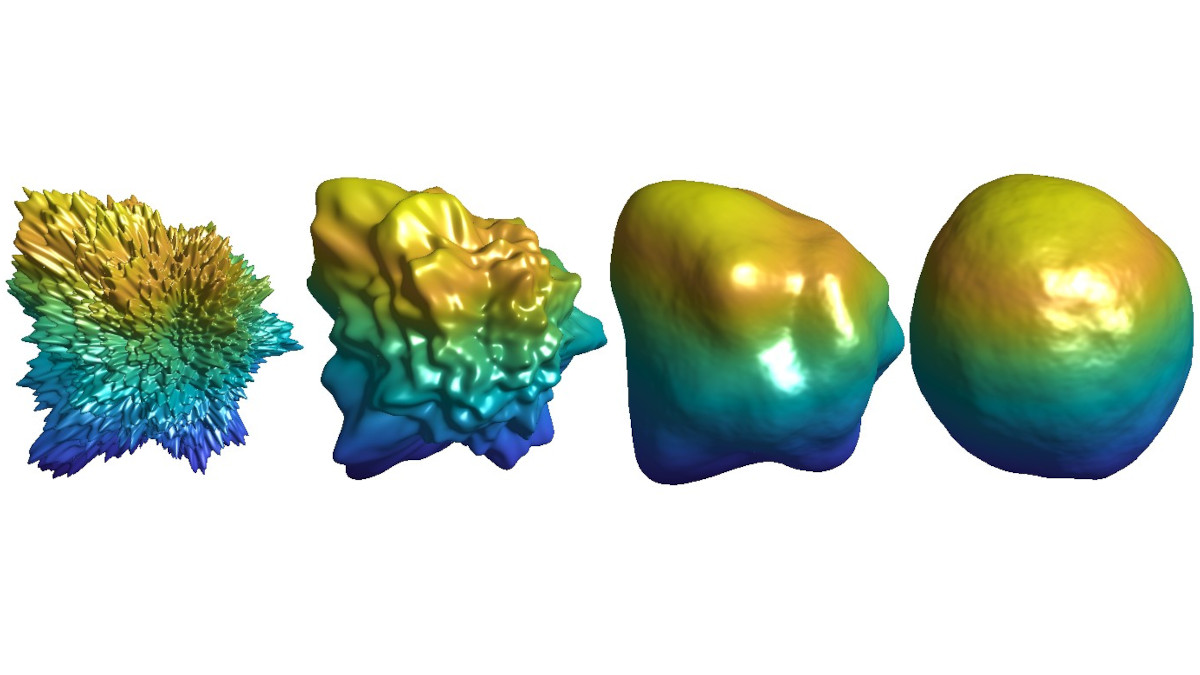# GENERATION OF RANDOM SURFACES BY STOCHASTIC PARTIAL DIFFERENTIAL EQUATIONSImagine a moving biological cell as recorded and published in a movie by Johns Hopkins Medicine
or an ice crystal in a cloud that starts to melt and freezes again as modeled for a fixed time in
[Nousiainen, McFarquhar 2004]. Both cases have in common that the surface is changing its shape
over time. In the ERC project Time-Evolving Stochastic Manifolds, we are trying to understand
the mathematics behind these examples and to find efficient methods to compute such
particles.

If we look at moving cells or ice crystals in clouds, then we observe that they usually appear in
very large numbers. They are looking and moving differently while having similar general properties
as the smoothness of the shape and its evolution in time. Generating big groups of these slightly
differing particles on a computer is infeasible with classical deterministic models such as partial
differential equations. We add a random component in order to allow for families of similar but
non-equal particles. Thus we need to simulate stochastic partial differential equations. Such
stochastic equations are used as models in physics and engineering whenever uncertainty is
involved. Including randomness is not restricted to our situation but necessary whenever
considering systems that are perturbed by the environment or that only allow for noisy
measurements. Although the deformation of particles involves in general more advanced
models based on nonlinear stochastic partial differential equations, we restrict ourselves
in what follows to the linear stochastic heat equation on the surface of a sphere, which
allows to give a first simple model for the change of shape of ice crystals in a cloud over
time.

The stochastic heat equation disturbed by noise through time can be rewritten as an infinite
system of stochastic ordinary differential equations that are the coefficients of a series expansion
with respect to the spherical harmonic functions—the Fourier basis on the sphere. In our linear
setting, we can solve all stochastic ordinary differential equations explicitly. Still we have infinitely
many solutions of the one-dimensional equations to be added to obtain the full solution. Since this is
impossible with a computer in finite time, we need to choose finitely many coefficients of our series
expansion. In numerical analysis this procedure is called a spectral approximation of
the solution to the stochastic heat equation. It is natural that the more terms of our
series we take the better the approximation gets, which is quantified in [Lang, Schwab 2015].

In most situations we are unable to solve the stochastic ordinary differential equations in the
series expansion explicitly. Then we need to approximate the time evolution by a method that
computes approximations recursively for small time steps. The standard procedure is to use the Euler–Maruyama approximation scheme which is the extension to the stochastic context of the
Euler time stepping method for ordinary differential equations. Combining finitely many terms in
the series expansion and such a time discretization, we obtain a fully discrete approximation of
the stochastic heat equation on the sphere that approximates the solution more and
more accurately the more terms of the series expansion we take and the smaller time
steps we use. This is shown in our recent preprint [Lang, Motschan-Armen 2023]. How
fast the approximation leads to the real solution depends on how smooth the initial
heat distribution and the disturbance by noise are. Our analysis of the quality of this
approximation shows that for a given regularity of the initial data and the noise, this spectral
Euler–Maruyama method gives optimal speed of convergence for the stochastic heat
equation on the sphere that matches earlier results known for equations in Euclidean
space.

Based on this described approximation, we simulate the solution to the stochastic heat equation
on the sphere and show one sample path evolving in time in the first video. The video starts with a
random initial condition mimicking later the initial state of the ice crystal. Over time the heat is
diffusing over the whole sphere with small fluctuations caused by the disturbance of the
noise.

Now we are ready to use this simulation to generate a toy model for the behavior of the shape of
an ice crystal in a cloud that starts to melt by the sun and freezes again while being continuously
disturbed by the environment. For this purpose we transform our simulated solution to a random surface in
the following way: Taking the exponential of our approximate solution, we obtain samples that are
strictly larger than zero and bounded. All points on the surface of the sphere have distance one from
the origin. Changing now this distance to the new value that is the exponential of our computed
solution, leads to the desired evolving surface. In the second video, we show the random surface
that corresponds to the solution of the stochastic heat equation on the sphere obtained
by this transformation. While the stochastic heat equation always aims at becoming a
perfect ball while being disturbed by noise at all times, a vibrating behavior becomes
visible if the stochastic wave equation on the sphere is used instead as shown in [Cohen, Lang 2022]. In the future we are planning to generalize this methodology to nonlinear
equations and to construct evolutions that are based on other shapes than spheres. This will
allow in the long run to simulate the example of families of moving cells in an efficient
way.